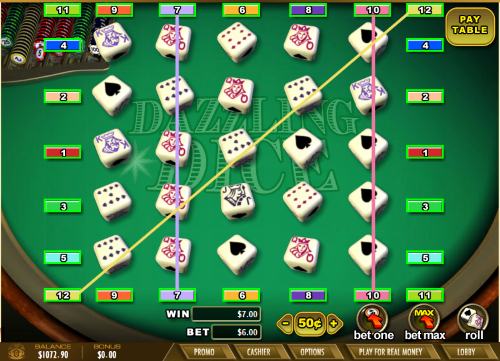## Wizard Recommends

Last Updated: October 8, 2014

# Dazzling Dice

## IntroductionDazzling Dice is a game of chance available at Internet casinos using Wager Gaming Technology software. The game is based on rolling 25 poker dice, arranged in a five by five matrix, and trying to form paying poker hands over the 12 pay lines.

## Rules

1. The player chooses a bet denomination, ranging from 25¢ to \$25, and then 1 to 12 pay lines.
2. The game uses poker dice. A poker die has on its six faces a 9, 10, jack, queen, king, and ace.
3. 25 poker dice will be rolled and arranged in a five by five matrix.
4. There are 12 lines running through the matrix, five vertical, five horizontal, and two diagonal.
5. The player is paid according to the poker value of each active pay line, according to the following pay table. All pays are on a "for one" basis.

### Pay Table

Hand Pays
Five of a kind 100
High straight 10
Low straight 8
Four of a kind 6
Full house 4
Three of a kind 2
Loser 0

## Analysis

The following table shows the number of combinations, probability, and return from each outcome. The lower right cell shows a return of 93.36%.

### Return Table

Hand Pays Permutations Probability Return
Five of a kind 100 6 0.000772 0.077160
High straight 10 120 0.015432 0.154321
Low straight 8 120 0.015432 0.123457
Four of a kind 6 150 0.019290 0.115741
Full house 4 300 0.038580 0.154321
Three of a kind 2 1,200 0.154321 0.308642
Loser 0 5,880 0.756173 0.000000
Total 7,776 1.000000 0.933642

## Strategy

The same casinos that offer this game should also offer Poker Dice, which a very similar game, but with just five dice and a more generous pay table returning 97.99%. Why settle for 93.4% when you can get 98.0%?

## Math Lesson

Since this is such a short page, I'll torture you with how to calculate the number of permutations of each winning hand.

• Five of a Kind: There are 6 combinations, one for each face of the dice.
• High Straight: There is only one type of high straight: A-K-Q-J-10. However, there are factorial(5) = 6×5×4×3×2 = 120 ways to arrange those five dice in order, since they are all different. So, 1×120=120 permutations.
• Low straight: A low straight is K-Q-J-10-9. The same odds as a high straight.
• Four of a Kind: There are 6 faces for the four of a kind and 5 left for the singleton. The singleton's position can be one of five places. So the total permutations are 6×5×5=150.
• Full House: There are 6 faces for the three of a kind and 5 left for the pair. There are combin(5,2)=10 possible positions in the five dice for the pair. So the total permutations are 6×5×10=300.
• Three of a Kind: There are 6 faces for the three of a kind and combin(5,2)=10 ways to choose the two singletons. There are combin(5,3)=10 possible positions in the five dice for the three of a kind. Then factorial(2) ways to position the two singletons in the remaining two positions. So the total permutations are 6×10×10×2=1200.

Written by: